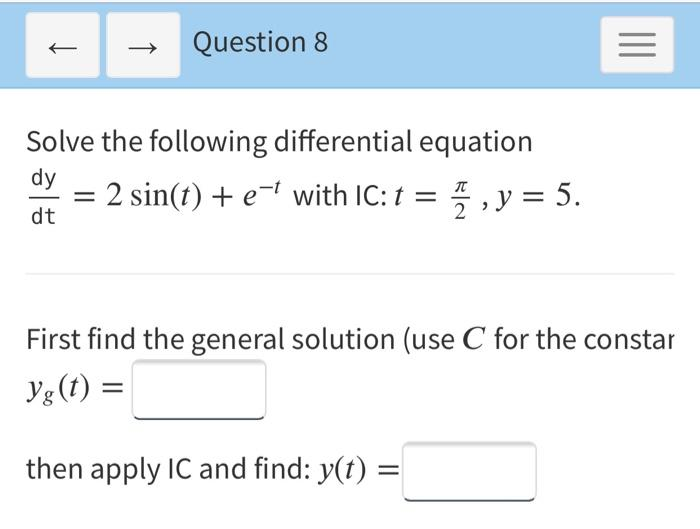### Create an Account

Already have account?

### Forgot Your Password ?

Home / Questions / + Question 8 = Solve the following differential equation dy = 2 sin(t) + e-l with IC: t = ...

# + Question 8 = Solve the following differential equation dy = 2 sin(t) + e-l with IC: t = 7 , y = 5. dt First find the general solution (use C for the constar Yg(t) = then apply IC and find: y(t) = =

+ Question 8 = Solve the following differential equation dy = 2 sin(t) + e-l with IC: t = 7 , y = 5. dt First find the general solution (use C for the constar Yg(t) = then apply IC and find: y(t) = =Apr 15 2021 View more View Less

#### Answer (Solved)Subscribe To Get Solution